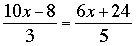SEARCH HOMEMath Central Quandaries & QueriesQuestion from Heidi: 10x-8 /3 =6x+24 /5.....I know the answer is 7/2 but how do I get there?Heidi,

When I seeI want to eliminate the fractions. 3 × 5 = 15 so multiply both sides by 15. The equation then becomes

5(10x - 8) = 3(6x + 24).

Simplify and solve for x.

If you need more help write back,
PennyMath Central is supported by the University of Regina and The Pacific Institute for the Mathematical Sciences.